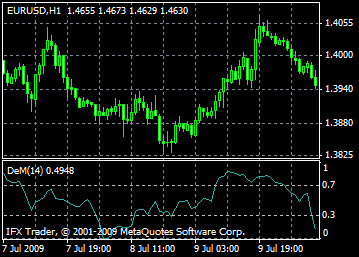# DeMarker - DeM

Technical Indicator DeMarker (DeM) is based on the comparison of the current and previous bars maximums. When the current maximum is situated above the previous one, the difference between them is registered. When the current maximum is situated at the same level with the previous one or above, zero volume is registered. Then, values received for a certain period of time are summed up, the result is a numeral indicator DeMarker which is divided by the same value plus the sum of differences between price minimums of the previous and current bars. When price minimum is above the previous level zero level is fixed.

When DeMarker falls below 30 the bullish price reversal expected. In case DeMarker indicator rises above 70 the bearish price reversal is expected.

The usage of the longer periods for calculations lets catch the long-term tendency in the market development. Indicators with the short periods let enter the market with the least risk and plan the moment of the deal making so that it will be in the tide of the main tendency.#### Calculation

The value of the DeMarker for the "i" interval is calculated as follows:

The DeMax(i) is calculated:

If high(i) > high(i-1) , then DeMax(i) = high(i)-high(i-1), otherwise DeMax(i) = 0

The DeMin(i) is calculated:

If low(i) < low(i-1), then DeMin(i) = low(i-1)-low(i), otherwise DeMin(i) = 0

The value of the DeMarker is calculated as:

DMark(i) = SMA(DeMax, N)/(SMA(DeMax, N)+SMA(DeMin, N))

Where:

SMA - Simple Moving Average;

N - the number of periods used in the calculation.

Tegye meg az első lépést célja felé
Nyisson számlát
Fejlessze kereskedési tudását pénzének kockáztatása nélkül
Nyisson demószámlát
Can't speak right now?